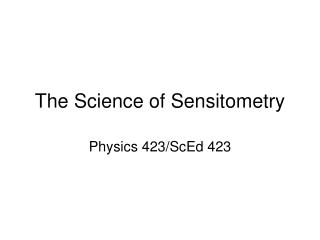DownloadDownload PresentationThe Science of Sensitometry

# The Science of Sensitometry

Télécharger la présentation## The Science of Sensitometry

- - - - - - - - - - - - - - - - - - - - - - - - - - - E N D - - - - - - - - - - - - - - - - - - - - - - - - - - -
##### Presentation Transcript

1. The Science of Sensitometry Physics 423/ScEd 423

2. Exactly what is film emulsion? The photographic emulsion can be compared to a chocolate nut bar. The nuts are suspended and separated by the chocolate as the silver halide grains are in the emulsion.

3. The Science of describing quantitatively the relationship between exposure and density for light-sensitive materials is called Sensitometry. Some Needed Terms: Intensity Transmittance Opacity Density Exposure

4. Intensity • A measure of what rate a source emits light • Was originally estimated as the standard candle • Thus candlepower is a unit of intensity • The unit is becoming replaced by the candela • Flux is the rate at which a source produces light in all directions (the unit is the lumen) • Flux is commonly used to measure flashlamp performance (e.g. a #11 flashbulb gives a peak output of 1,800,000 lumens) • Quantity of light may be given as lumen-seconds (e.g. a #11 flashbulb is rated at 28,000 lumen-seconds)

5. A piece of film Opacity is defined as the Light Absorbing Quality and is the reciprocal of the Transmittance. Density is the logarithm of the Opacity. What is the “logarithm”. Use your calculator to find out. Exposure = Intensity of the Light (foot candles) times exposure time (seconds)

6. An Example • Suppose the light passing through a region in a negative is 50% (i.e. only 50% of the light makes it through). • Then the Transmittance is 0.5 • The Opacity is 1/.5 or 2 • The density is Log 2 = 0.3

7. The parts of a negative can be measured with a densitometer to determine actual density measurements A Negative with density equivalents

8. Putting Density and Exposure together = The Characteristic Curve or the H&D Curve For each exposure, 0.3 is a stop (a halving or doubling) of exposure

9. Summary of Sensitometry, the science of measuring film response to exposure and development • As long as the film exposure is placed on the straight line portion of the characteristic curve you will get a good picture. • If the film is underexposed the shadows will line under the toe and thus rendering no printable shadow detail (when in doubt, overexpose). • The steeper the characteristic curve, the higher the film contrast. • The toe is where small amounts of exposure first register density. • Characteristic curves vary according to the film type and development.

10. An example of three different film types Which film is faster? Slower? Has more contrast?